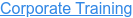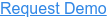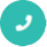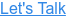#### Digital Marketing# Interview Questions For Advanced MS Excel

by Bhavya Sri, on May 23, 2017 5:21:41 PM## Q1. What is Microsoft Excel?

Ans: Microsoft Excel is an electronic spreadsheet application that enables users to store, organize, calculate and manipulate the data with formulas using a spreadsheet system broken up by rows and columns. It also provides the flexibility to use an external database to do analysis, make reports, etc. thus saving lots of time.

## Q2. What is ribbon?

Ans: Ribbon refers to the topmost area of the application that contains menu items and toolbars available in MS-Excel. Ribbon can be shown/hidden using CTRL+F1. The ribbon runs on the top of the application and is the replacement for the toolbars and menus.  The ribbons have various tabs on the top, and each tab has its own group of commands.

## Q3. Explain Spreadsheet and its Basics.

Ans: Spreadsheet can be compared to a paper ledger sheet. It consists or rows and columns and their intersection called cells.

## Q4. How many data formats are available in Excel? Name some of them.

Ans: Eleven data formats are available in Microsoft Excel for data Storage. Example:

• Number – Stores data as a number
• Currency – Stores data in the form of currency
• Date – Data is stored as dates
• Percentage – Stores numbers as a percentage
• Text Formats – Stores data as string of texts

## Q5. Specify the order of operations used for evaluating formulas in Excel.

Ans: The order of operations in Microsoft Excel is same as in standard mathematics. It’s defined by the term “PEMDAS” or “BEDMAS”.

• Parentheses or Brackets
• Exponent
• Multiplication
• Division
• Subtraction

## Q6. How can you wrap the text within a cell?

Ans: You must select the text you want to wrap, and then click wrap text from the home tab and you can wrap the text within a cell.

## Q7. Explain Macro in MS-Excel.

Ans: Macros are used for iterating over a group of tasks. Users can create macros for their customized repetitive functions and instructions. Macros can be either written or recorded depending on the user.

## Q8. Which are the two macro languages in MS-Excel?

Ans: XLM and VBA (Visual Basic Applications).  Earlier versions of Excel used XLM. VBA was introduced in Excel 5 and mostly used now.

## Q9. Is it possible to prevent someone from copying the cell from your worksheet?

Ans: Yes, it is possible. To protect your worksheet from getting copied, you need to go into Menu bar >Review > Protect Sheet > Password.  By entering a password, you can secure your sheet from getting copied by others.

## Q10. What are charts in MS-Excel?

Ans: To enable graphical representation of the data in Excel, charts are provided. A user can use any chart type, including column, bar, line, pie, scatter, etc. by selecting an option from Insert tab’s Chart group.## Q11. How can you sum up the Rows and Column number quickly in the Excel sheet?

Ans: By using SUM function, you can get the total sum of the rows and columns, in an Excel worksheet.

## Q12. Explain few useful functions in Excel.

Ans: Following are the functions available in Excel for manipulating the data:

• Math and Financial Functions – SQRT, DEGREE, RAND(), GCD
• Logical Functions – IF, AND, FALSE, TRUE
• Date and Time functions – NOW(), DATEVALUE(), WEEKDAY(NOW())
• Index Match – VLOOKUP and INDEX MATCH
• Pivot tables

## Q13. What does a red triangle at the top right of a cell indicate?

Ans: The red triangle indicates that some comment is associated with the cell. Hover the mouse over it, and you can read the full comment.

## Q14. How can you add a new Excel worksheet?

Ans: To add a new Excel worksheet, you should insert worksheet tab at the bottom of the screen.

## Q15. What is the use of NameBox in MS-Excel?

Ans: Name Box is used to return to a particular area of the worksheet by typing the range name or cell address in the name box.

## Q16. How can you resize the column?

Ans: To resize the column, you should change the width of one column and then drag the boundary on the right side of the column heading till the width you want.  The other way of doing it is to select the Format from the home tab, and in Format you have to select AUTOFIT COLUMN WIDTH under cell section. On clicking on this, the cell size will get formatted.

## Q17. Explain pivot tables and its uses.

Ans: A pivot table is a tool that allows for quick summarization of large data. It automatically performs a sort, count, total or average of the data stored in the spreadsheet and displays result in another spreadsheet. It saves a lot of time. Allows to link external data sources to our Excel.

## Q18. What are three report formats that are available in Excel?

Following are the types of report formats:

• Compact
• Report
• Tabular

## Q19. How would you provide a Dynamic range in “Data Source” of Pivot Tables?

Ans: To provide a dynamic range in “Data Source” of Pivot tables, first, create a named range using offset function and base the pivot table using a named range created in the first step.

## Q20. Is it possible to make Pivot table using multiple sources of data?

Ans: If the multiple sources are different worksheets, from the same workbook, then it is possible to make Pivot table using multiple sources of data.

## Q21. Which event do you use to check whether the Pivot Table is modified or not?

Ans: To check whether the pivot table is modified or not we use “PivotTableUpdate” in worksheet containing the pivot table.

## Q22. How can you disable automatic sorting in pivot tables?

Ans: To disable automatic sorting in pivot tables:

Go To > More Sort Options > Right Click ‘Pivot tables’ > Select ‘sort menu’ > select ‘More Options’ > deselect ‘Sort automatically’.

## Q23. What is Freeze Panes in MS-Excel?

Ans: To lock any row or column, freeze panes is used. The locked row or column will be visible on the screen even after we scroll the sheet vertically or horizontally.

## Q24. What could you do to stop the pivot table from loosing the column width upon refreshing?

Ans: Format loss in a pivot table can be stopped simply by changing the pivot table options.  Under the “Pivot Table Options” turn on the “Enable Preserve Formatting” and disable “Auto Format” option.

## Q25 Explain workbook protection types in Excel.

Ans: Excel provides three ways to protect a workbook:

• Password protection for opening a workbook
• Protection for adding, deleting, hiding and unhiding sheets
• Protection from changing size or position of windows.

## Q26. Explain the difference between SUBSTITUTE and REPLACE function in MS-Excel?

Ans: The SUBSTITUTE function substitutes one or more instances of old text with the new text in a string.

Syntax: SUBSTITUTE(text, oldText, newText, [instanceNumber])

Example: Let text at A2 be Guru99,Guru99

SUBSTITUTE(A2,”9″,”8″,1) =>Guru89,Guru99

SUBSTITUTE(A2,”9″,”8″,2) =>Guru88,Guru99

SUBSTITUTE(A2,”9″,”9″) =>Guru88,Guru88

The REPLACE function swaps part of the text string with another set of text.

Syntax: REPLACE(oldText, startNumber, NumberCharacters, newText)

Example: Let text at A2 be Guru99

REPLACE(A2,5,1,”00″) =>Guru009

## Q27. Difference between COUNT, COUNTA, COUNTIF and COUNTBLANK in Ms-Excel.

Ans: COUNT is used to count cells containing numbers, dates, etc. any value stored as number excluding blanks.

COUNTA or Count All is used to count any cell value containing numbers, text, logical values, etc. any type of value excluding blanks.

COUNTBLANK count blank cells or cells with an empty string.

COUNTIF and COUNTIFS count cells matching a certain criteria.

## Q28. What is IF function in Excel?

Ans: To perform the logic test IF function is performed. It checks whether certain conditions is true or false. If the condition is true, then it will give result accordingly if the condition is false then the result or out-put will be different.

Example: For example, you select the cell, and you want to display that cell as “Greater than five,” when value is true (=5 or 5) and “less than five” when value is false (<5). For that by using IF condition you can display result.

=IF (Logical test, value if true, value if false)

=IF (A1>5, “Greater than five, “Less than five”)

## Q29. Can we create shortcuts to Excel functions?

Ans: Yes. ‘Quick Access Toolbar’ above the home button can be customized to display most frequently used shortcuts.

## Q30. What is the use of LOOKUP function in Excel?

Ans: In Microsoft Excel, the LOOKUP function returns a value from a range or an array.

## Q31. How can you apply the same formatting to every sheet in a workbook in MS-Excel?

Ans: Right Click ‘Worksheet tab’ > Choose ‘Select All Sheets’. Now any formatting done will be applied to the whole workbook. To apply to a particular group of sheets, select only those sheets that need formatting.

## Q32. What are left, right, fill and distributed alignments?

Ans: Left /Right alignment align the text to left and right most of the cell.

Fill as the name suggests, fill the cell with same text repetitively.

Distributed, spread the text across the width of the cell.

## Q33. To move to the previous worksheet and next sheet, what keys will you press?

Ans: To move to the previous worksheet, you will use the keys Ctrl + PgUp, and to move to the next sheet you will use keys Ctrl + PgDown.

## Q34. What filter will you use, if you want more than two conditions or if you want to analyze the list using database function?

Ans: You will use Advanced Criteria Filter, to analyze the list or if more than two conditions should be tested.

## Q35. What is the quick way to return to a particular area of a worksheet?

Ans: The quick way to return to a specific area of the worksheet is by using name box.  You can type the cell address or range name in name box to return to a specific area of a worksheet.

## Q36. Which function is used to determine the day of the week for a date?

Ans: WEEKDAY () returns the day of the week for a particular date counting from Sunday.

Example: Let date at A1 be 12/30/2016

WEEKDAY(A1,1) =>6

## Q37. What is the benefit of using formula in Excel sheet?

Ans: Calculating the numbers in Excel sheet, not only help you to give the final ‘sum up’ of the number but, it also calculates automatically the number replaced by another number or digit.  Through Excel sheet, the complex calculations become easy like payroll deduction or averaging the student’s result.

## Q38. What is the “What If” condition in Excel formulas?

Ans: The “What If” condition is used to change the data in Microsoft Excel formulas to give different answers.

Example: You are buying a new car and want to calculate the exact amount of tax that will be levied on it then you can use the “What If” function.  For instance, there are three cells A4,B4, and C4. First cell says about the amount, the second cell will tell about the percentage (7.5%) of tax and the final cell will calculate the exact amount of tax.

## Q39. How can you disable the automating sorting in pivot tables?

Ans: To disable the automating sorting in pivot tables,

Go to > “More Sort Options”> Right Click “Pivot table” > Select “Sort” menu > Select “More Options” > Deselect the “Sort automatically when the report is created.”

## Q40. What is the AND function does in Excel?

Ans: Like IF function, AND function also does the logical function. To check whether the output will be true or false the AND function will evaluate at least one mathematical expression located in another cell in the spreadsheet. If you want to see the output of more than one cells in a single cell, it is possible by using AND function.

Example: If you have two cells, A1 and A2, and the value you put in those two cells are >5 and you want result should display as ‘TRUE’ in cell B1 if value>5, and ‘False’ if any of those values<5. You can use AND function to do that.

## Q41. How cell reference is useful in the calculation?

Ans: In order to avoid writing the data again and again for calculating purpose, cell reference is used. When you write any formula, for specific function, you need to direct Excel the specific location of that data. This location is referred as, cell reference. So, every time a new value added to the cell, the cell will calculate according to the reference cell formula.